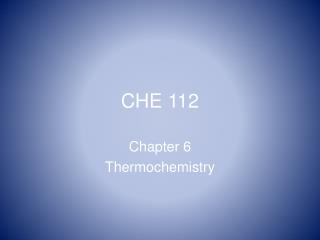Download PresentationCHE 112CHE 112 - PowerPoint PPT Presentation

Download PresentationCHE 112
An Image/Link below is provided (as is) to download presentation

Download Policy: Content on the Website is provided to you AS IS for your information and personal use and may not be sold / licensed / shared on other websites without getting consent from its author. While downloading, if for some reason you are not able to download a presentation, the publisher may have deleted the file from their server.

- - - - - - - - - - - - - - - - - - - - - - - - - - - E N D - - - - - - - - - - - - - - - - - - - - - - - - - - -
Presentation Transcript

1. CHE 112 Chapter 6 Thermochemistry

2. Course Introduction • Prerequisites • Course Policy and Grading • Homework • Shopping List • Lab Start up

3. Chapter 6 Overview • Energy, work and heat • 1st Law of Thermodynamics • Enthalpy, H

4. Energy • Defined: capacity to do work • PE (composition or location) • KE = ½ m v 2 3 types of molecular motion • work = f x d ( where f = ma ) work and energy have SAME units, joules![algebraic proof in text]

5. Systems • System vs surroundings • Three types of systems open - matter and energy exchange closed - only energy exchanged isolated – no energy /matter exchanged

6. Internal Energy, U • U defined: total energy withinsystem KE and PE molecular motion, KE bonding, PE • Heat, “q” transferred energy (joules) • Work, w = - P Δ V • State functions

7. 1st Law Δ U = q + w Sign Conventions [very impt] + energy absorbed by systemq > 0 heat absorbed from surroundings w > 0 work done ON system [compression] - energy lost by systemq < 0 heat given off by system to surroundings w< 0 work done by system [ expansion] see example 6.1B page 223

8. Terms • Adiabatic [ thermal insulation], q =0 • Exothermic • Endothermic

9. Che Reactions • @ V = constant w = 0 So, ΔU = qv • @ P = constant ΔU = qp + w = qp - P ΔV • Or qp = ΔU + P ΔV

10. EnthalpyH = U + PV • @ P = constant, qp = ΔH = ΔU + PΔV • ΔH extensive a state function qp = ΔH, unique value ; “heat of rxn” • Recall, exo and endo • Enthalpy Diagrams or Energy Profiles

11. ΔH and Stoichiometry Example: Given H2 + Cl2 → 2 HCl ΔH = - 184 KJfind ΔH if a) 12.8 g H 2 react b) 6.25 mol. HCl form

12. Heat Capacity and Specific Heat • C = q / ΔT mass dependent ( extensive) • Intensive molar C or SH • SH = q / m ΔT q = SH x mass x ΔT • RE: lab determination of SH of a metal [ p. 233 ex. 6.9 ]

13. Hess’ Law of Heat Summation A + B → C + D ΔH1 C + B → E + F ΔH2 ___________________________________ A + 2B → D + E + F ΔHrxnΔHrxn = ΔH1 +ΔH2 Example page 237 sec 6.6

14. Standard Enthaply of FormationΔHf0 • Define: standard state • “standard heat of formation” • ΔHf0 = 0 for elements • See table 6.2 page 240 ΔHrxn0 = ∑ ΔHp0 - ∑ ΔHr0 using molar coeffsexample 6.15 page 241

15. End Ch 6 • You may skip sec 6.8 • Homework questions? logoff